TitleCollege Algebra Tutorial 2: Integer Exponents

WTAMU > Virtual Math Lab > College AlgebraLearning Objectives

 After completing this tutorial, you should be able to: Use the definition of exponents. Simplify exponential expressions involving multiplying like bases, zero as an exponent, dividing like bases, negative exponents, raising a base to two exponents, raising a product to an exponent and raising a quotient to an exponent.Introduction

 This tutorial covers the basic definition and rules of exponents.  The rules it covers are the product rule, quotient rule, power rule, products to powers rule, quotients to powers rule, as well as the definitions for zero and negative exponents. Exponents are everywhere in algebra and beyond.  Let's see what we can do with exponents.Tutorial

 Definition of Exponents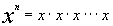(note there are n  x's in the product) x = base,    n = exponent

 Exponents are another way to write multiplication. The exponent tells you how many times a base appears in a PRODUCT.Example 1: Evaluate.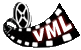View a video of this example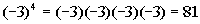*Write the base -3 in a product 4 times *MultiplyExample 2: Evaluate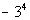.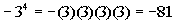*Negate 3 to the fourth *Put a - in front of 3 written in a product 4 times *Multiply

 Hey, this looks a lot like example 1!!!!  It may look alike, but they ARE NOT exactly the same.  Can you see the difference between the two??  Hopefully, you noticed that in example 1, there was a ( ) around the - and the 3.  In this problem, there is no ( ).  This means the - is NOT part of the base, so it will not get expanded like it did in example 1.  It is interpreted as finding the negative or opposite of 3 to the fourth power.Example 3: Evaluate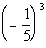.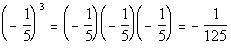*Write the base -1/5 in a product 3 times *Multiply

 Multiplying Like Bases With Exponents (The Product Rule for Exponents) Specific Illustration

 Let’s first start by using the definition of exponents to help you to understand how we get to the law for multiplying like bases with exponents:Note that 2 + 3 = 5, which is the exponent we ended up with.  We had 2 x’s written in a product plus another 3 x’s written in the product for a total of 5 x’s in the product.  To indicate that we put the 5 in the exponent.   Let's put this idea together into a general rule:

 Multiplying Like Bases With Exponents (Product Rule for Exponents) in general,In other words, when you multiply like bases you add your exponents. The reason is, exponents count how many of your base you have in a product.  So if you are continuing that product, you are adding on to the exponents.Example 4:   Use the product rule to simplify the expression.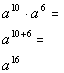*When mult. like bases you add your exponentsExample 5:   Use the product rule to simplify the expression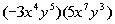.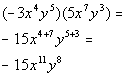*When mult. like bases you add your exponents

 Zero as an exponent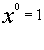Except for 0, any base raised to the 0 power simplifies to be the number 1. Note that the exponent doesn’t become 1, but the whole expression simplifies to be the number 1.Example 6:  Evaluate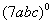.*Any expression raised to the 0 power simplifies to be 1Example 7:  Evaluate.

 Be careful on this example.  Order of operations says to evaluate exponents before doing any multiplication.  This means we need to find x raised to the 0 power first and then multiply it by -15.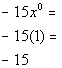*x raised to the 0 power is 1 *Multiply

 Dividing Like Bases With Exponents (Quotient Rule for Exponents) Specific Illustration

 Let’s first start by using the definition of exponents to help you to understand how we get to the law for dividing like bases with exponents: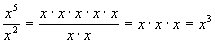Note how 5 - 2 = 3, the final answer’s exponent.  When you multiply you are adding on to your exponent, so it should stand to reason that when you divide like bases you are taking away from your exponent. Let's put this idea together into a general rule:

 Dividing Like Bases With Exponents (Quotient Rule for Exponents) in general,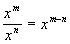In other words, when you divide like bases you subtract their exponents. Keep in mind that you always take the numerator’s exponent minus your denominator’s exponent, NOT the other way around.Example 8:  Find the quotient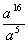.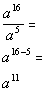*When div. like bases you subtract your exponentsExample 9:  Find the quotient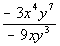.*When div. like bases you subtract your exponents

 Negative Exponents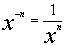or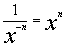Be careful with negative exponents.  The temptation is to negate the base, which would not be a correct thing to do. Since exponents are another way to write multiplication and the negative is in the exponent, to write it as a positive exponent we do the multiplicative inverse which is to take the reciprocal of the base.Example 10:  Simplify.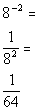*Rewrite with a pos. exp. by taking recip. of base    *Use def. of exponents to evaluateExample 11:  Simplify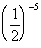.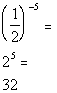*Rewrite with a pos. exp. by taking recip. of base *Use def. of exponents to evaluate

 Base Raised to Two Exponents (Power Rule for Exponents) Specific Illustration

 Let’s first start by using the definition of exponents as well as the law for multiplying like bases to help you to understand how we get to the law for raising a base to two exponents: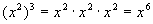Note how 2 times 3 is 6, which is the exponent of the final answer.   We can think of this as 3 groups of 2, which of course would come out to be 6.

 Base Raised to two Exponents (Power Rule for Exponents) in general,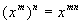In other words, when you raise a base to two exponents, you multiply those exponents together. Again, you can think of it as n groups of m if it helps you to remember.Example 12:   Simplify.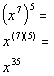*When raising a base to two powers you mult. your exponentsExample 13:   Simplify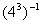.*When raising a base to two powers you mult. your exponents *Use the definition of neg. exponents to rewrite as the recip. of base *Use the def. of exponents to evaluate

 A Product Raised to an Exponent (Products to Powers Rule for Exponents) Specific Illustration

 Let’s first start by using the definition of exponents to help you to understand how we get to the law for raising a product to an exponent:Note how both bases of your product ended up being raised by the exponent of 3.

 A Product Raised to an Exponent (Products to Powers Rule for Exponents) in general,In other words, when you have a PRODUCT (not a sum or difference) raised to an exponent, you can simplify by raising each base in the product to that exponent.Example 14:   Simplify.*When raising a product to an exponent, raise each base of the product to that exponentExample 15:   Simplify.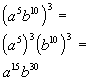*When raising a product to an exponent, raise each base of the product to that exponent *Mult. exponents when using power rule for exponents

 A Quotient Raised to an Exponent (Quotients to Powers Rule for Exponents) Specific Illustration

 Let’s first start by using the definition of exponents to help you to understand how we get to the law for raising a quotient to an exponent: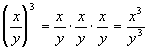Since division is really multiplication of the reciprocal, it has the same basic idea as when we raised a product to an exponent.

 A Quotient Raised to an Exponent (Quotients to Powers Rule for Exponents) in general,In other words, when you have a QUOTIENT (not a sum or difference) raised to an exponent, you can simplify by raising each base in the numerator and denominator of the quotient to that exponent.Example 16:   Simplify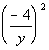.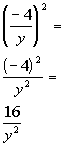*When raising a quotient to an exponent, raise each base of the quotient to that exponent *Use def. of exponents to evaluate

 Of course, we all know that life isn’t so cut and dry.  A lot of times you need to use more than one definition or law of exponents to get the job done.  What we did above was to set the foundation to make sure you have a good understanding of the different ideas associated with exponents.  Next we will work through some problems which will intermix these different laws.

 Simplifying an Exponential Expression

 When simplifying an exponential expression,  write it so that each base is written one time with one POSITIVE exponent.  In other words, write it in the most condensed form you can making sure that all your exponents are positive. A lot of times you have to use more than one rule to get the job done.  As long as you use the rule appropriately you should be fine.Example 17:    Simplify the exponential expression.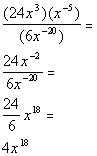*When div. like bases you subtract your exponents: -2 - (-20) = 18Example 18:     Simplify the exponential expression.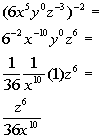Example 19:    Simplify the exponential expression.Be careful going into the last line.   Since b doesn't have a negative exponent, we DO NOT take the reciprocal of b.  The other bases each have a negative exponent, so those bases we have to take the reciprocal of.Practice Problems

 These are practice problems to help bring you to the next level.  It will allow you to check and see if you have an understanding of these types of problems. Math works just like anything else, if you want to get good at it, then you need to practice it.  Even the best athletes and musicians had help along the way and lots of practice, practice, practice, to get good at their sport or instrument.  In fact there is no such thing as too much practice. To get the most out of these, you should work the problem out on your own and then check your answer by clicking on the link for the answer/discussion for that  problem.  At the link you will find the answer as well as any steps that went into finding that answer.Practice Problems 1a - 1f: Simplify the exponential expression.

 1a.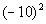(answer/discussion to 1a) 1b.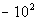(answer/discussion to 1b)

 1c.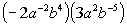(answer/discussion to 1c) 1d.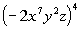(answer/discussion to 1d)

 1e.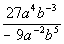(answer/discussion to 1e) 1f.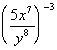(answer/discussion to 1f)Need Extra Help on these Topics?

The following are webpages that can assist you in the topics that were covered on this page:

 http://www.wtamu.edu/academic/anns/mps/math/mathlab/int_algebra/int_alg_tut23_exppart1.htm This website gives the definition of and some of the basic rules for exponents.  http://www.sosmath.com/algebra/logs/log2/log2.html#shortcuts This webpage gives the definition of exponents. http://www.wtamu.edu/academic/anns/mps/math/mathlab/int_algebra/int_alg_tut24_exppart2.htm This website helps you with some of the basic rules for exponents. http://www.purplemath.com/modules/exponent.htm This webpage gives an overall review of exponents.  http://www.ltcconline.net/greenl/courses/152A/polyExp/intexp.htm This webpage goes over the rules of exponents. http://www.sosmath.com/algebra/logs/log3/log31/log31.html This website helps you with the product rule for exponents. http://www.sosmath.com/algebra/logs/log3/log32/log32.html This website helps you with the quotient rule for exponents. http://www.sosmath.com/algebra/logs/log3/log33/log33.html This website helps you with the rule for raising a base to two exponents.

Go to Get Help Outside the Classroom found in Tutorial 1: How to Succeed in a Math Class for some more suggestions.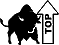Videos at this site were created and produced by Kim Seward and Virginia Williams Trice.

Last revised on Feb. 15, 2008 by Kim Seward.